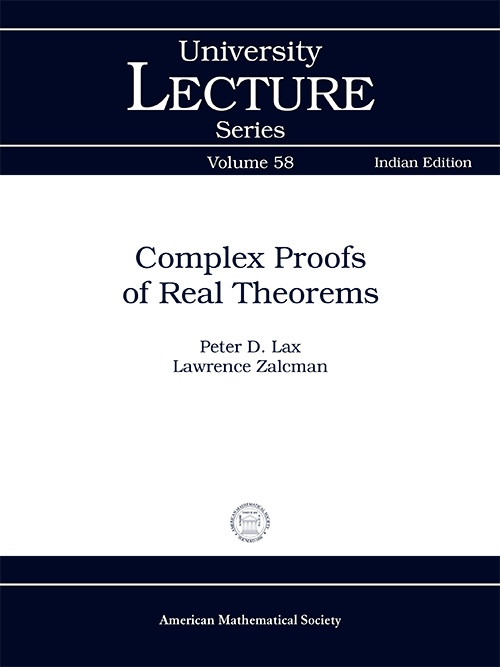## Complex Proofs of Real Theorems

#### About the Book

This is an extended meditation on Hadamard’s famous dictum, “The shortest and best way between two truths of the real domain often passes through the imaginary one,” directed at an audience acquainted with analysis at the first year graduate level. It aims at illustrating how complex variables can be used to provide quick and efficient proofs of a wide variety of important results in such areas of analysis as approximation theory, operator theory, harmonic analysis, and complex dynamics. Topics discussed include weighted approximation on the line, Müntz’s theorem, Toeplitz operators, Beurling’s theorem on the invariant spaces of the shift operator, prediction theory, the Riesz convexity theorem, the Paley–Wiener theorem, the Titchmarsh convolution theorem, the Gleason–Kahane–Zelazko theorem, and the Fatou–Julia–Baker theorem. The discussion begins with the world’s shortest proof of the fundamental theorem of algebra and concludes with Newman’s almost effortless proof of the prime number theorem. Four brief appendices provide all necessary background in complex analysis beyond the standard first year graduate course. Lovers of analysis and beautiful proofs will read and reread this slim volume with pleasure and profit.

#### Contributors (Author(s), Editor(s), Translator(s), Illustrator(s) etc.)

Peter D Lax is professor at the Department of Mathematics, Courant Institute of Mathematical Sciences, New York University, New York, USA.

Lawrence Zalcman is Professor Emeritus at the Department of Mathematics, Bar-Ilan University, Ramat Gan, Israel.

#### Table of Contents

Preface
Chapter 1. Early Triumphs
Chapter 2. Approximation
Chapter 3. Operator Theory
Chapter 4. Harmonic Analysis
Chapter 5. Banach Algebras: The Gleason–Kahane–Zelazko Theorem
Chapter 6. Complex Dynamics: The Fatou–Julia–Baker Theorem
Chapter 7. The Prime Number Theorem Coda: Transonic Airfoils and SLE
Appendix A. Liouville’s Theorem in Banach Spaces
Appendix B. The Borel–Carathéodory Inequality
Appendix C. Phragmén–Lindelöf Theorems
Appendix D. Normal Families

`